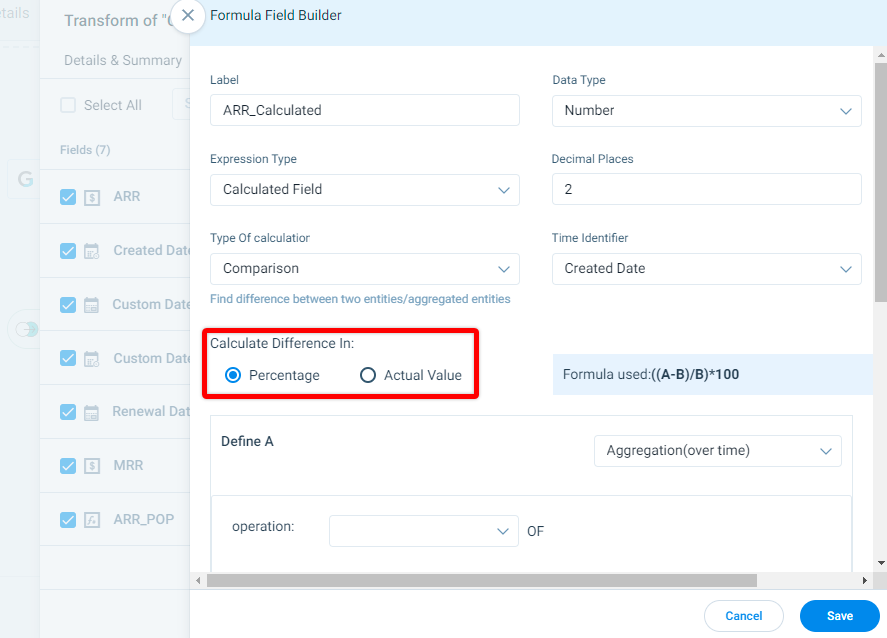# Use Calculated Fields in Data Designer

## Overview

Calculated Fields is used to perform two types of calculations, Aggregation and Comparison over a period of time. This function is available as an option while configuring formula fields in the Data Transform phase of Data Designer.

Add a Calculated field in Data Designer to perform the following:

• Create a new field that is a rolling average of the last 7 days of a usage measure.
• You can create a field that is a comparison of aggregated usage measures over the last 7 days versus prior 30 days.

## How To Add Calculated Field

1. Navigate to the Preparation stage in Data Designer.
2. Apply Transform on a dataset. A new window with the dataset name Transform appears.
3. In the Fields tab, click Add Formula Field. The Formula Field Builder window appears.
4. Perform the following actions:
1. In the Label textbox, enter the unique name of the formula.
2. From the Data Type dropdown, select Number as the data type.
3. From the Expression Type dropdown, select Calculated Field.
4. In the Decimal Places textbox, enter the decimal places to round the value of the metric to be measured.
5. From the Time Identifier dropdown, select the date field for the comparison to be made.
6. From the Type of Calculator dropdown, select either of the following calculation type:
• Comparison: Used to calculate a comparison between two measures or numeric fields.
• Aggregation (over time):  Used to aggregate a measure over a period of time. (Example: Count of cases in the last month).

### Calculation Type as Comparison

When Comparison is set as Calculation Type, the difference between two entities or aggregated entities is calculated.Choose either of the following methods to calculate the difference:

• Percentage: ((A-B) / B) *100
• Actual Value: A - B#### Define A

In Define A configuration:

1. Select one of the following:
• Aggregation(over time)
1. From the Operation dropdown, select an aggregation type (Sum, Average, Count, Count distinct, Min or Max) and the measure on which the aggregation should be applied.
2. From the Timeframe dropdown, select the date range in which this calculation should be executed. For example: past 2 days or past 4 weeks or past 3 months.
3. Select Adjust for missing data for the aggregation type Average to skip counting the time period if the data is missing for day/week/month. Example: If you choose to do an average of the past 4 weeks and there is no data for one week,the data for that one week will be skipped.
• Select a Field
1. From the dropdown, select a fixed metric value added in the field.

#### Define B

In Define B configuration:

• Aggregation(over time)
1. From the Operation dropdown, select an aggregation type (Sum, Average, Count, Count distinct, Min or Max) and the measure on which the aggregation should be applied.
2. From the Timeframe dropdown, select one of the following options:
• Past: Completed period in the past.
• Prior: Period that immediately precedes the past days/weeks/months selected in A.
• Same Period: Same period as in N days/weeks/months as selected in A.
3. Select Adjust for missing data for the aggregation type Average to skip counting the time period if the data is missing for day/week/month. Example: If you choose to do an average of the past 4 weeks and there is no data for one week,the data for that one week will be skipped.
• Select a Field
1. From the dropdown, select a fixed metric value added in the field.

### Calculation Type as Aggregation(over time)

In the Aggregation type, calculate the aggregation of a metric over a time period.

1. From the Operation dropdown, select an aggregation type (Sum, Average, Count, Count distinct, Min or Max) and the measure on which the aggregation should be applied.
2. From the Timeframe dropdown, select the date range in which this calculation should be executed. For example: past 2 days or past 4 weeks or past 3 months.
3. Select Adjust for missing data for the aggregation type Average to skip counting the time period if the data is missing for day/week/month. Example: If you choose to do an average of the past 4 weeks and there is no data for one week, the data for that one week will be skipped.